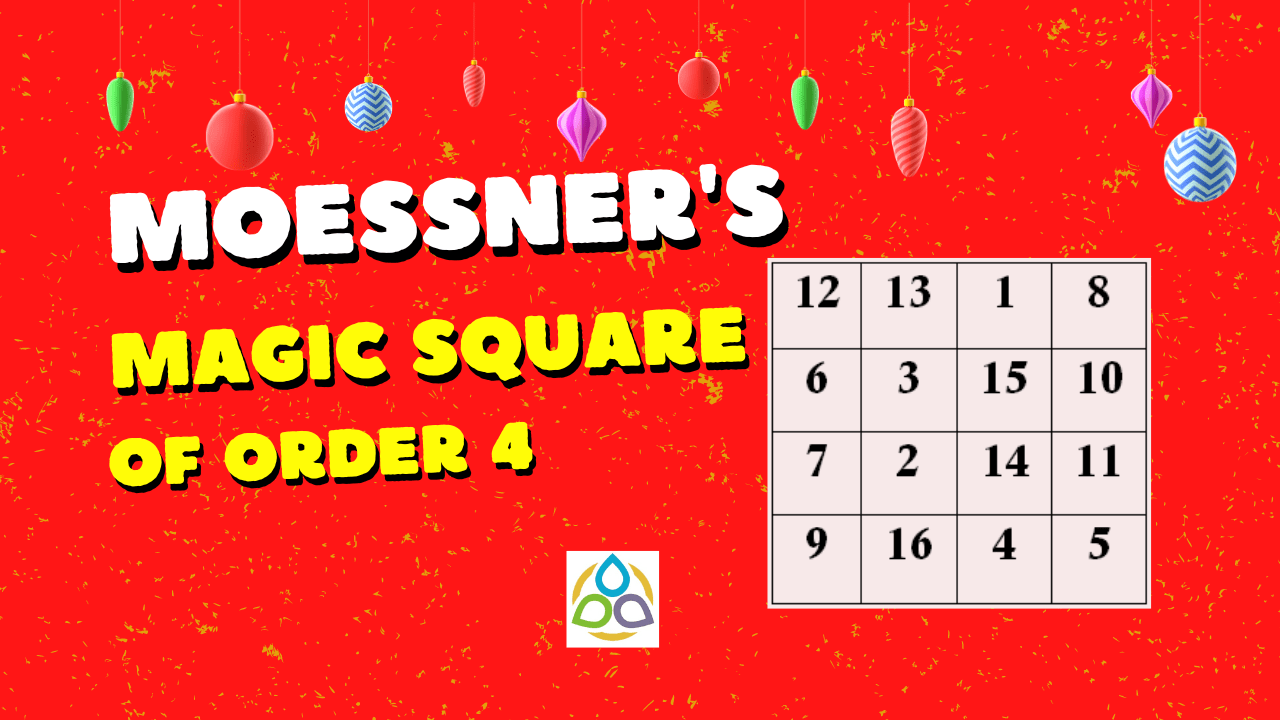## Moessner’s Magic Square of Order 4

Anyone who cannot cope with mathematics is not fully human. At best he is a tolerable subhuman who has learned to wear shoes, bathe, and not make messes in the house.

Robert A. HEINLEIN

Welcome to the blog Math1089 – Mathematics for All.

I’m glad you came by. I wanted to let you know I appreciate your spending time here on the blog very much. I do appreciate your taking time out of your busy schedule to check out Math1089!

Numbers are beautiful, but arranging them in a particular way is more interesting. In this blog post, we will consider the first sixteen natural numbers and arrange them in a particular way to see the beauties in them. In fact, we will arrange them in a square to see the beauties of number relations.

A magic square is an arrangement of n2 numbers into an n × n square array such that:

• the sum of the elements of each row;
• the sum of the elements in each column;
• the sum of the elements along each diagonal

are the same. When the elements are not specified, it is conventional for them to be the first n2 (strictly) positive integers. Consider the following magic square of order 4 due to Moessner.

Following the definition of a magic square, we have

the sum of the elements of each row are same:

12 + 13 + 1 + 8 = 34;

6 + 3 + 15 + 10 = 34;

7 + 2 + 14 + 11 = 34;

9 + 16 + 4 + 5 = 34.

the sum of the elements of each column are same:

12 + 6 + 7 + 9 = 34;

13 + 3 + 2 + 16 = 34;

1 + 15 + 14 + 4 = 34;

8 + 10 + 11 + 5 = 34.

the sum of the elements along each diagonal are same:

12 + 3 + 14 + 5 = 34;

8 + 15 + 2 + 9 = 34.

In addition to the above properties, the following are a few additions.

The sums of the cubes of the entries on the diagonals of Moessner’s order 4 magic square are equal.

123 + 33 + 143 + 53

= 1728 + 27 + 2744 + 125

= 4624;

and

93 + 23 + 153 + 83

= 729 + 8 + 3375 + 512

= 4624

Your suggestions are eagerly and respectfully welcome! See you soon with a new mathematics blog that you and I call Math1089 – Mathematics for All!“.